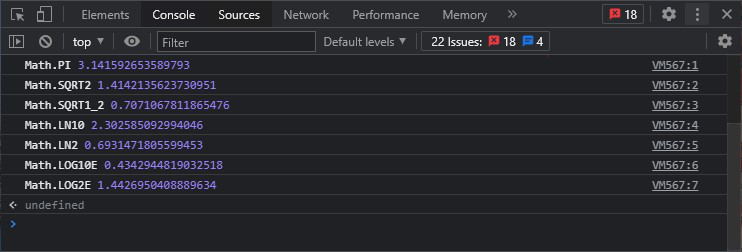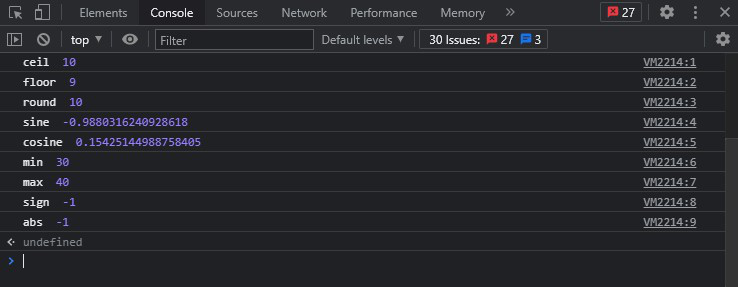# What is the use of Math object in JavaScript ?

• Difficulty Level : Easy
• Last Updated : 27 Aug, 2021

Math is an inbuilt object that has attributes and methods for mathematical functions and constants. It’s not a function object. Math object works with the Number type. The Math object does not have a constructor. All properties and methods of Math are fixed/static. The cosine function is known as Math.cos(y) while the constant pi is known as Math.PI, where y is the method’s argument. All the properties and methods of Math are static and can be called by using Math as an object without creating it. In this article, we will discuss various available methods & properties used in Javascript. We will start with the Math properties in Javascript.

Static Math Properties: The Math properties & their descriptions are listed below:

Syntax:

`Math.property`

Example:

## HTML

 ` ` `<``html` `lang``=``"en"``> ` `  ``<``head``> ` `    ``<``meta` `charset``=``"UTF-8"` `/> ` `    ``<``meta` `http-equiv``=``"X-UA-Compatible"`  `          ``content``=``"IE=edge"` `/> ` `    ``<``meta` `name``=``"viewport"`  `          ``content="``width``=``device``-width,  ` `                   ``initial-scale``=``1``.0" /> ` `    ``<``title``>Document ` `  `` ` `   `  `  ``<``body``> ` `    ``<``script``> ` `      ``console.log("Math.PI", Math.PI); ` `      ``console.log("Math.SQRT2", Math.SQRT2); ` `      ``console.log("Math.SQRT1_2", Math.SQRT1_2); ` `      ``console.log("Math.LN10", Math.LN10); ` `      ``console.log("Math.LN2", Math.LN2); ` `      ``console.log("Math.LOG10E", Math.LOG10E); ` `      ``console.log("Math.LOG2E", Math.LOG2E); ` `    `` ` `  `` ` ``

Output:Static Math Methods: The methods associated with the Math object are listed below, along with their descriptions.

Syntax:

`Math.method(number)`

Example:

## HTML

 ` ` `<``html` `lang``=``"en"``> ` `  ``<``head``> ` `    ``<``meta` `charset``=``"UTF-8"` `/> ` `    ``<``meta` `http-equiv``=``"X-UA-Compatible"`  `          ``content``=``"IE=edge"` `/> ` `    ``<``meta` `name``=``"viewport"`  `          ``content="``width``=``device``-width,  ` `                   ``initial-scale``=``1``.0" /> ` `    ``<``title``>Document ` `  `` ` `  ``<``body``> ` `    ``<``script``> ` `      ``console.log("ceil ", Math.ceil(9.6)); ` `      ``console.log("floor ", Math.floor(9.6)); ` `      ``console.log("round ", Math.round(9.6)); ` `      ``console.log("sine ", Math.sin(30)); ` `      ``console.log("cosine ", Math.cos(30)); ` `      ``console.log("min ", Math.min(30, 40)); ` `      ``console.log("max ", Math.max(30, 40)); ` `      ``console.log("sign ", Math.sign(-40)); ` `      ``console.log("abs ", Math.sign(-40)); ` `    `` ` `  `` ` ``

Output:My Personal Notes arrow_drop_up
Recommended Articles
Page :